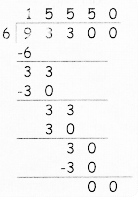Students can download 5th Maths Term 1 Chapter 2 Numbers Ex 2.8 Questions and Answers, Notes, Samacheer Kalvi 5th Maths Guide Pdf helps you to revise the complete Tamilnadu State Board New Syllabus, helps students complete homework assignments and to score high marks in board exams.

## Tamilnadu Samacheer Kalvi 5th Maths Solutions Term 1 Chapter 2 Numbers Ex 2.8

Question 1.
Find quotient and remainder.
a. 5732 ÷ 9
b. 4735 ÷ 5
c. 3032 ÷ 7
d. 43251 ÷ 10
e. 2532 ÷ 4
a. 5732 ÷ 9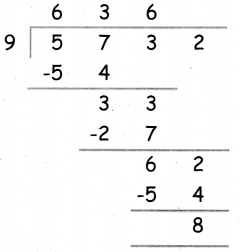Quotient = 636
Remainder = 8b. 4735 ÷ 5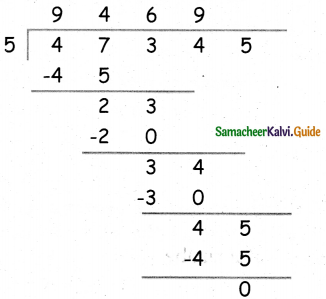Quotient = 9469
Remainder = 0

c. 3032 ÷ 7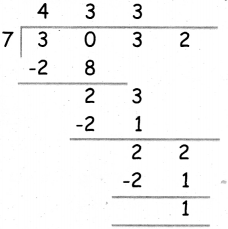Quotient = 433
Remainder = 1d. 43251 ÷ 10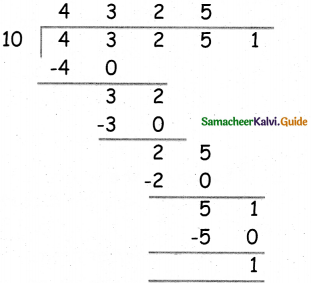Quotient = 4325
Remainder = 1

e. 2532 ÷ 4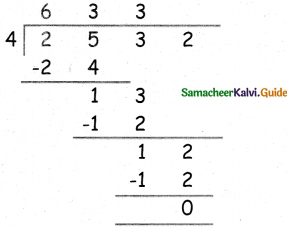Quotient = 633
Remainder = 0Question 2.
a. 3057 families are living in a town. The town panchayat decided to split the town into 3 wards. How many families will be there in each panchayat?
Total number of families in a town = 3057
No. of wards = 3
∴ Total number of families in each ward
= 3057 ÷ 3 = 1,019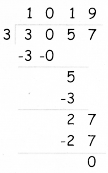b. A water board distributes 28,049 litres daily to a town in 7 lorries. How much of water will each lorry carry?
Total liters of water = 28,049
No. of lorries = 7
∴ Water is got by each lorries
= 28049 ÷ 7
= 4,007 litres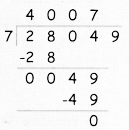c. A company gives ₹ 93,300 as salary for 6 workers equally. How much salary will each worker get?